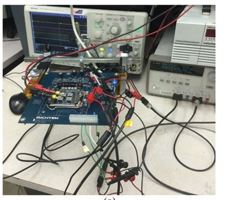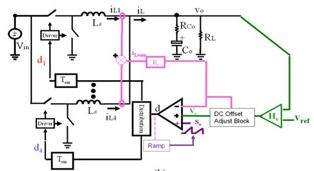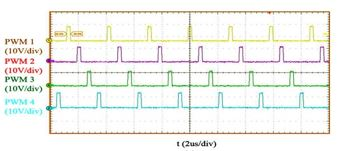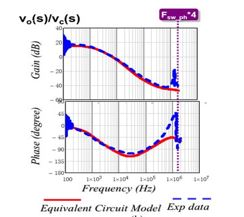Contact Us
LIBRARY

# A Three-Terminal Switch Model of Constant On-Time Current Mode With External Ramp CompensationFig. 1. (a) Experimental setup.
Multiphase constant on-time current-mode control based on a pulse distribution structure is widely used in voltage regulator applications for microprocessors. To minimize the ripple cancellation effect, external ramp compensation is used in commercial products. However, using an external ramp will introduce dynamics to the system, and if this is not taken into consideration, the stability margin will suffer. We propose a simple equivalent circuit model based on a three-terminal switch concept, which considers the effect of the external ramp by adding an additional R - L branch. The equivalent circuit model can be reduced to a previous unified three-terminal switch model when the external ramp is zero, and can be reduced to a model of constant on-time voltage mode control when the external ramp is much larger than the inductor current ramp. The proposed three-terminal switch model is a complete model, which can be used to examine all transfer functions, and is accurate up to half of the switching frequency. The model is verified by SIMPLIS simulation and experimental measurement.Fig. 1. (b) Simplified diagram for RT8859M Demo Board.Fig 2. (a) Experimental verification for case II: D = 0.1 (Vin = 12 V, Vo = 1V): Steady-state waveformFig. 2. (b) Verification of control-to-output voltage transfer function.
INDUSTRY PARTNERS